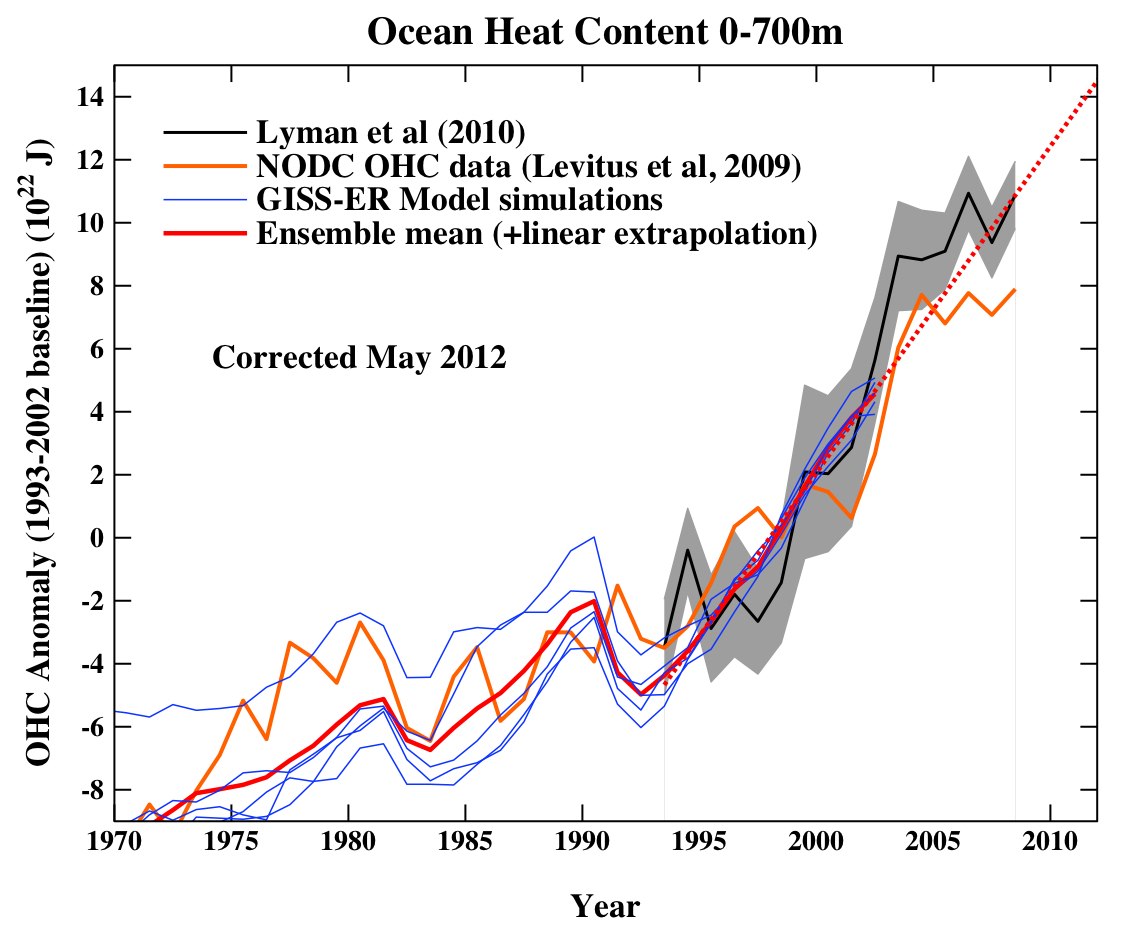## Friday, May 28, 2010

### Ocean heat content, and useful unitsThe recent post at realclimate about measured increases in ocean heat content has an interesting graph whose y-axis is labelled in spectacular units, 1022 J. Even exajoules are not that big! (1EJ = 1018 J)...
One comment on that blog suggested it would be good to re-express in other units that are more familiar - say degrees Celsius, or watts per square metre.
Here goes...
The graph shows the ocean heat content increasing by about 20 x 1022 J in 40 years.
First, let's express the change in heat content as a average rise in temperature of the top 700 metres of the ocean (which is what was actually measured to make these graphs!).
`Temperature rise = (Heat content increase) / (Volume of water) /                         (Heat capacity)                 = 20 x 1022 J /                    (350 x 106 km2 * 700 m) /                    (4.2 x 106 J/K/m3)                 = 0.19 K (or 0.19 degrees C).`

Second, let's express the rate of increase in heat content in terms of a net power per unit area required.
`Power per unit area = (Heat content increase) / Time / Area                    = 20 x 1022 J / (40 years) /                       (350 x 106 km2)                    = 0.45 W/m2.`

This can be compared with other things measured in the same units - see for example pages 20 and 170 in Sustainable Energy – without the hot air.
Hope this helps!

## Sunday, May 9, 2010

### SEWTHA online - Index addedI've added an alphabetical index page to the html edition of Sustainable Energy - without the hot air. I hope this helps! This index is identical to the version in the paper edition of the book, except that the page-numbers are clickable hypertext links.